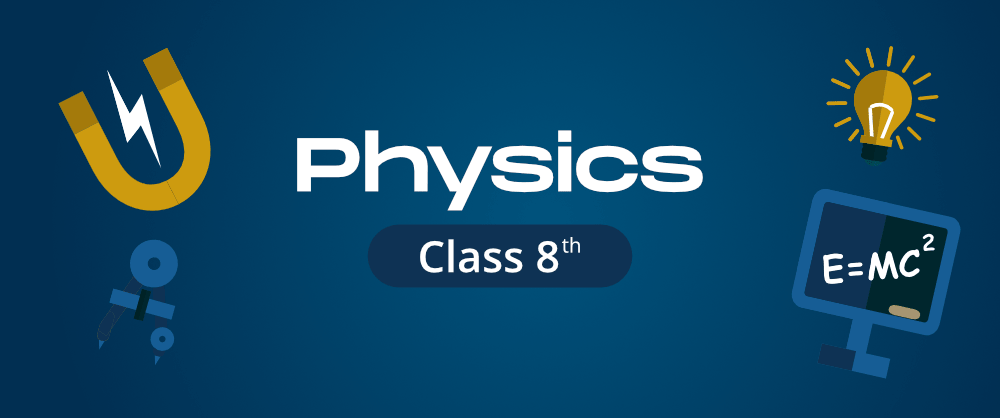CBSE Physics Class 8 Notes

• Last Updated : 11 Nov, 2021

GeeksforGeeks came up with a unique outlook to School Learning by providing CBSE Class 8 Physics notes. Since science is an integral subject of School Learning so is Physics too. Physics is one of the disciplines that most students grow up studying. This subject, in general, offers an understanding of how the fundamental elements of our daily lives function and perform. Therefore, GeeksforGeeks Class 8 Physics Notes ensures that you study everything in accordance with your NCERT textbook, core books, or reference books. The following chapter-wise CBSE Class 8 Physics notes consist of 7 chapters including Force and Pressure, Sound, Light, etc which are further equipped with various concepts, everyday life examples, and numericals on the same, in order to understand and solve the problems in a better way.Chapter 1: Force and Pressure

The first chapter of Physics in the Science curriculum of Class 8 is Force and Pressure. Force is simply the push and pulls upon an object while Pressure is defined as the force applied per unit area of the object. Both the terms Force and Pressure are the basic building blocks of mechanics (a part of Physics). This chapter consists of two parts Force and Pressure respectively, Force includes basic concepts, types, properties/characteristics of forces, Friction and its different types, etc. While, the section on Pressure comprises Basic concepts, Types of Pressure and Buoyancy, etc which are explained in the articles listed below:

Chapter 2: Sound

Sound is the second chapter of Physics in the Science curriculum of Class 8. Sound is something that can be heard from anything like Television, aeroplane, etc. Scientifically, Sound can be defined as an energy form that propagates in the form of waves and is produced by vibrations. The topics covered in this chapter helps to understand, the basic concept of Sound, Production, and propagation of Sound, Characteristics of Sound waves (like Amplitude, Time period, Frequency, Pitch, and Loudness), Types of Sounds (Audible and Inaudible Sounds) and about the Pollution caused by excessive sound that is Noise pollution. All such topics are thoroughly explained under the following headings as listed below:

Chapter 3: Chemical Effects of Electric Current

The third chapter of Physics in the Science textbook comes up is called Chemical Effects of Electric Current. Electric Current is the rate of the flow of electrons or charges in a conductor. Therefore, the passage of electric current through a conducting solution results in chemical reactions. This chapter builds a basic idea of electricity, which will be studied in later classes. As this chapter helps to develop a general idea, so we will learn about electroplating and the chemical changes that occur when electricity flows through the liquid and its applications. The topic discussed are listed below:

Chapter 4: Some Natural Phenomena

Some Natural Phenomena is the fourth chapter of Class 8 Physics. As the name of the chapter says, Some Natural Phenomena, therefore, it explains some naturally causing phenomena like lightning, earthquake which are destructive to some extent. Thus, the topics covered in this chapter come up with the basic idea of Lightening and Earthquakes, the destruction caused by them, how they occur? and measures to be taken during them. These concepts are discussed in the following articles:

Chapter 5: Light

The fifth chapter in the Class 8 Physics syllabus is Light. Light is a form of energy that enables us to see our surroundings when it gets reflected from objects around us. Light is emitted from a source such as the Sun. The term usually refers to visible light, which is visible to the human eye and is responsible for the sense of sight. This chapter helps to develop the general and the basic concept of Optics (a branch of Physics). According to the CBSE Class 8 Science Curriculum, here we have covered topics like the concept of Reflection, Laws of Reflection, Types of Reflection, Structure, and Working of the Human Eye, Dispersion of Light, etc. are discussed in the following articles listed below:

Chapter 6: Stars and the Solar System

The last chapter of CBSE Class 8 Physics is Stars and the Solar System that leads one to go to infinity and beyond. Topics covered are celestial objects, Sun, comets, astronomy, planets, meteors, and meteorites, moon, satellites, meteor shower, stars, Astronomy in ancient India, artificial satellites, constellation, Mangalyaan which are discussed in the following articles listed below:

My Personal Notes arrow_drop_up